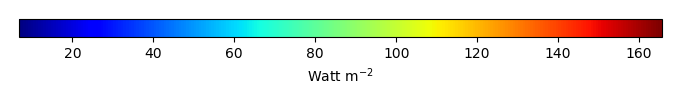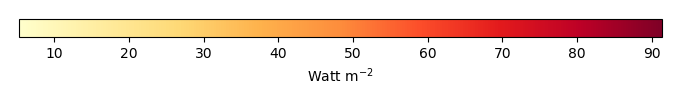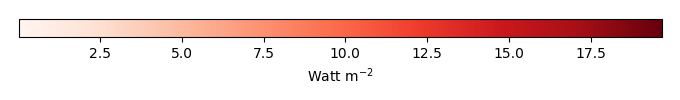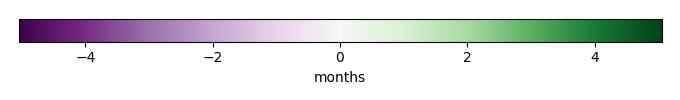# Mean State

Period Mean (original grids) [Watt m-2]
Model Period Mean (intersection) [Watt m-2]
Model Period Mean (complement) [Watt m-2]
Benchmark Period Mean (intersection) [Watt m-2]
Benchmark Period Mean (complement) [Watt m-2]
Bias [Watt m-2]
RMSE [Watt m-2]
Phase Shift [months]
Bias Score 
RMSE Score 
Seasonal Cycle Score 
Spatial Distribution Score 
Interannual Variability Score 
Overall Score 
Benchmark [-] 38.3
CRUNCEPv7 [-] 35.9 35.5 39.6 15.5 -3.45 11.0 1.68 0.346 0.380 0.729 0.936 0.443 0.536
GSWP3v1 [-] 33.8 33.5 39.6 15.5 -5.43 11.5 1.57 0.300 0.376 0.750 0.970 0.507 0.546
WFDEI [-] 31.5 31.2 39.6 15.5 -7.79 13.9 2.18 0.230 0.334 0.631 0.938 0.503 0.495
Period Mean (original grids) [Watt m-2]
Model Period Mean (intersection) [Watt m-2]
Model Period Mean (complement) [Watt m-2]
Benchmark Period Mean (intersection) [Watt m-2]
Benchmark Period Mean (complement) [Watt m-2]
Bias [Watt m-2]
RMSE [Watt m-2]
Phase Shift [months]
Bias Score 
RMSE Score 
Seasonal Cycle Score 
Spatial Distribution Score 
Interannual Variability Score 
Overall Score 
Benchmark [-] 52.3
CRUNCEPv7 [-] 47.2 47.7 52.8 14.1 -5.16 13.2 1.29 0.442 0.397 0.842 0.986 0.468 0.589
GSWP3v1 [-] 45.9 46.4 52.8 14.1 -6.56 13.5 1.32 0.404 0.383 0.835 0.990 0.496 0.582
WFDEI [-] 46.0 46.6 52.8 14.1 -6.27 15.4 1.39 0.388 0.333 0.825 0.970 0.493 0.557
Period Mean (original grids) [Watt m-2]
Model Period Mean (intersection) [Watt m-2]
Model Period Mean (complement) [Watt m-2]
Benchmark Period Mean (intersection) [Watt m-2]
Benchmark Period Mean (complement) [Watt m-2]
Bias [Watt m-2]
RMSE [Watt m-2]
Phase Shift [months]
Bias Score 
RMSE Score 
Seasonal Cycle Score 
Spatial Distribution Score 
Interannual Variability Score 
Overall Score 
Benchmark [-] 39.3
CRUNCEPv7 [-] 36.1 36.1 40.4 16.6 -4.22 12.0 1.79 0.372 0.421 0.729 0.923 0.483 0.558
GSWP3v1 [-] 36.1 36.1 40.4 16.6 -4.29 12.1 1.88 0.369 0.398 0.710 0.918 0.531 0.554
WFDEI [-] 36.4 36.4 40.4 16.6 -3.94 12.5 1.99 0.369 0.374 0.698 0.969 0.542 0.555
Period Mean (original grids) [Watt m-2]
Model Period Mean (intersection) [Watt m-2]
Model Period Mean (complement) [Watt m-2]
Benchmark Period Mean (intersection) [Watt m-2]
Benchmark Period Mean (complement) [Watt m-2]
Bias [Watt m-2]
RMSE [Watt m-2]
Phase Shift [months]
Bias Score 
RMSE Score 
Seasonal Cycle Score 
Spatial Distribution Score 
Interannual Variability Score 
Overall Score 
Benchmark [-] 31.6
CRUNCEPv7 [-] 39.6 39.5 31.5 32.6 9.02 22.2 0.253 0.726 0.542 0.983 0.984 0.757 0.756
GSWP3v1 [-] 37.2 37.2 31.5 32.6 6.18 20.2 0.280 0.773 0.549 0.980 0.867 0.805 0.754
WFDEI [-] 41.4 41.3 31.5 32.6 10.4 27.4 0.210 0.694 0.458 0.985 0.984 0.767 0.725
Period Mean (original grids) [Watt m-2]
Model Period Mean (intersection) [Watt m-2]
Model Period Mean (complement) [Watt m-2]
Benchmark Period Mean (intersection) [Watt m-2]
Benchmark Period Mean (complement) [Watt m-2]
Bias [Watt m-2]
RMSE [Watt m-2]
Phase Shift [months]
Bias Score 
RMSE Score 
Seasonal Cycle Score 
Spatial Distribution Score 
Interannual Variability Score 
Overall Score 
Benchmark [-] 23.5
CRUNCEPv7 [-] 28.5 28.5 25.9 15.5 3.10 10.9 2.16 0.401 0.382 0.659 0.198 0.421 0.407
GSWP3v1 [-] 26.9 26.9 25.9 15.5 1.64 11.1 1.56 0.336 0.366 0.771 0.222 0.540 0.433
WFDEI [-] 24.9 24.9 25.9 15.5 -0.337 11.3 1.68 0.292 0.359 0.748 0.346 0.500 0.434
Period Mean (original grids) [Watt m-2]
Model Period Mean (intersection) [Watt m-2]
Model Period Mean (complement) [Watt m-2]
Benchmark Period Mean (intersection) [Watt m-2]
Benchmark Period Mean (complement) [Watt m-2]
Bias [Watt m-2]
RMSE [Watt m-2]
Phase Shift [months]
Bias Score 
RMSE Score 
Seasonal Cycle Score 
Spatial Distribution Score 
Interannual Variability Score 
Overall Score 
Benchmark [-] 21.4
CRUNCEPv7 [-] 25.2 25.0 21.5 17.6 3.49 10.2 0.512 0.751 0.537 0.938 0.880 0.671 0.719
GSWP3v1 [-] 23.8 23.7 21.5 17.6 2.19 10.4 0.647 0.793 0.512 0.923 0.924 0.720 0.731
WFDEI [-] 24.1 23.9 21.5 17.6 2.44 11.9 0.496 0.761 0.471 0.944 0.798 0.699 0.691
Period Mean (original grids) [Watt m-2]
Model Period Mean (intersection) [Watt m-2]
Model Period Mean (complement) [Watt m-2]
Benchmark Period Mean (intersection) [Watt m-2]
Benchmark Period Mean (complement) [Watt m-2]
Bias [Watt m-2]
RMSE [Watt m-2]
Phase Shift [months]
Bias Score 
RMSE Score 
Seasonal Cycle Score 
Spatial Distribution Score 
Interannual Variability Score 
Overall Score 
Benchmark [-] 30.0
CRUNCEPv7 [-] 38.7 38.6 30.5 14.9 7.41 13.8 1.05 0.559 0.444 0.861 0.949 0.643 0.650
GSWP3v1 [-] 35.0 34.9 30.5 14.9 3.75 11.5 0.980 0.671 0.468 0.869 0.961 0.666 0.684
WFDEI [-] 38.4 38.3 30.5 14.9 7.00 16.2 1.20 0.583 0.376 0.834 0.863 0.678 0.618
Period Mean (original grids) [Watt m-2]
Model Period Mean (intersection) [Watt m-2]
Model Period Mean (complement) [Watt m-2]
Benchmark Period Mean (intersection) [Watt m-2]
Benchmark Period Mean (complement) [Watt m-2]
Bias [Watt m-2]
RMSE [Watt m-2]
Phase Shift [months]
Bias Score 
RMSE Score 
Seasonal Cycle Score 
Spatial Distribution Score 
Interannual Variability Score 
Overall Score 
Benchmark [-] 29.5
CRUNCEPv7 [-] 36.6 36.6 30.6 14.2 5.15 12.0 0.893 0.564 0.411 0.901 0.970 0.459 0.619
GSWP3v1 [-] 32.7 32.7 30.6 14.2 1.54 11.6 0.981 0.572 0.397 0.889 0.980 0.500 0.623
WFDEI [-] 32.6 32.6 30.6 14.2 1.33 12.8 1.06 0.535 0.363 0.882 0.929 0.498 0.595
Period Mean (original grids) [Watt m-2]
Model Period Mean (intersection) [Watt m-2]
Model Period Mean (complement) [Watt m-2]
Benchmark Period Mean (intersection) [Watt m-2]
Benchmark Period Mean (complement) [Watt m-2]
Bias [Watt m-2]
RMSE [Watt m-2]
Phase Shift [months]
Bias Score 
RMSE Score 
Seasonal Cycle Score 
Spatial Distribution Score 
Interannual Variability Score 
Overall Score 
Benchmark [-] 33.0
CRUNCEPv7 [-] 39.6 39.4 32.8 37.1 7.10 22.6 0.279 0.777 0.556 0.981 0.957 0.693 0.753
GSWP3v1 [-] 35.8 35.6 32.8 37.1 3.16 18.9 0.279 0.833 0.582 0.981 0.823 0.758 0.760
WFDEI [-] 38.9 38.6 32.8 37.1 6.21 25.4 0.167 0.795 0.493 0.989 0.934 0.720 0.737
Period Mean (original grids) [Watt m-2]
Model Period Mean (intersection) [Watt m-2]
Model Period Mean (complement) [Watt m-2]
Benchmark Period Mean (intersection) [Watt m-2]
Benchmark Period Mean (complement) [Watt m-2]
Bias [Watt m-2]
RMSE [Watt m-2]
Phase Shift [months]
Bias Score 
RMSE Score 
Seasonal Cycle Score 
Spatial Distribution Score 
Interannual Variability Score 
Overall Score 
Benchmark [-] 35.1
CRUNCEPv7 [-] 42.4 42.4 36.7 14.1 4.32 10.8 0.616 0.573 0.545 0.953 0.966 0.616 0.700
GSWP3v1 [-] 41.4 41.5 36.7 14.1 3.44 10.5 0.452 0.590 0.542 0.962 0.959 0.636 0.705
WFDEI [-] 42.5 42.6 36.7 14.1 4.40 11.6 0.586 0.581 0.492 0.955 0.977 0.706 0.700
Period Mean (original grids) [Watt m-2]
Model Period Mean (intersection) [Watt m-2]
Model Period Mean (complement) [Watt m-2]
Benchmark Period Mean (intersection) [Watt m-2]
Benchmark Period Mean (complement) [Watt m-2]
Bias [Watt m-2]
RMSE [Watt m-2]
Phase Shift [months]
Bias Score 
RMSE Score 
Seasonal Cycle Score 
Spatial Distribution Score 
Interannual Variability Score 
Overall Score 
Benchmark [-] 19.8
CRUNCEPv7 [-] 25.8 25.8 20.7 10.0 4.62 10.7 0.995 0.650 0.539 0.893 0.962 0.645 0.705
GSWP3v1 [-] 21.6 21.6 20.7 10.0 0.867 9.68 1.06 0.718 0.545 0.881 0.825 0.646 0.693
WFDEI [-] 23.4 23.4 20.7 10.0 2.45 11.6 1.38 0.696 0.480 0.815 0.959 0.679 0.685
Period Mean (original grids) [Watt m-2]
Model Period Mean (intersection) [Watt m-2]
Model Period Mean (complement) [Watt m-2]
Benchmark Period Mean (intersection) [Watt m-2]
Benchmark Period Mean (complement) [Watt m-2]
Bias [Watt m-2]
RMSE [Watt m-2]
Phase Shift [months]
Bias Score 
RMSE Score 
Seasonal Cycle Score 
Spatial Distribution Score 
Interannual Variability Score 
Overall Score 
Benchmark [-] 23.6
CRUNCEPv7 [-] 47.5 47.5 43.1 14.1 3.57 16.6 0.876 0.586 0.488 0.889 0.880 0.545 0.646
GSWP3v1 [-] 44.9 44.9 43.1 14.1 0.998 14.3 0.866 0.626 0.497 0.886 0.877 0.595 0.663
WFDEI [-] 47.5 47.5 43.1 14.1 3.63 18.7 0.981 0.572 0.440 0.868 0.892 0.605 0.636
Period Mean (original grids) [Watt m-2]
Model Period Mean (intersection) [Watt m-2]
Model Period Mean (complement) [Watt m-2]
Benchmark Period Mean (intersection) [Watt m-2]
Benchmark Period Mean (complement) [Watt m-2]
Bias [Watt m-2]
RMSE [Watt m-2]
Phase Shift [months]
Bias Score 
RMSE Score 
Seasonal Cycle Score 
Spatial Distribution Score 
Interannual Variability Score 
Overall Score 
Benchmark [-] 64.3
CRUNCEPv7 [-] 81.4 81.2 66.2 16.5 12.9 20.9 0.649 0.529 0.552 0.939 0.982 0.534 0.681
GSWP3v1 [-] 78.4 78.2 66.2 16.5 10.1 18.6 0.505 0.561 0.576 0.953 0.985 0.543 0.699
WFDEI [-] 82.8 82.6 66.2 16.5 14.3 22.1 0.741 0.502 0.548 0.922 0.977 0.532 0.671
Period Mean (original grids) [Watt m-2]
Model Period Mean (intersection) [Watt m-2]
Model Period Mean (complement) [Watt m-2]
Benchmark Period Mean (intersection) [Watt m-2]
Benchmark Period Mean (complement) [Watt m-2]
Bias [Watt m-2]
RMSE [Watt m-2]
Phase Shift [months]
Bias Score 
RMSE Score 
Seasonal Cycle Score 
Spatial Distribution Score 
Interannual Variability Score 
Overall Score 
Benchmark [-] 34.3
CRUNCEPv7 [-] 43.2 43.2 34.2 35.0 10.5 25.3 0.373 0.690 0.540 0.970 0.963 0.691 0.732
GSWP3v1 [-] 39.0 38.9 34.2 35.0 5.70 19.5 0.221 0.775 0.583 0.983 0.959 0.796 0.780
WFDEI [-] 44.9 44.9 34.2 35.0 12.1 30.1 0.268 0.674 0.464 0.980 0.953 0.770 0.718
Period Mean (original grids) [Watt m-2]
Model Period Mean (intersection) [Watt m-2]
Model Period Mean (complement) [Watt m-2]
Benchmark Period Mean (intersection) [Watt m-2]
Benchmark Period Mean (complement) [Watt m-2]
Bias [Watt m-2]
RMSE [Watt m-2]
Phase Shift [months]
Bias Score 
RMSE Score 
Seasonal Cycle Score 
Spatial Distribution Score 
Interannual Variability Score 
Overall Score 
Benchmark [-] 24.8
CRUNCEPv7 [-] 33.8 33.8 27.0 14.3 6.34 10.8 0.818 0.512 0.453 0.914 0.838 0.495 0.611
GSWP3v1 [-] 33.8 33.8 27.0 14.3 6.38 10.9 0.849 0.528 0.444 0.914 0.900 0.550 0.630
WFDEI [-] 34.3 34.3 27.0 14.3 6.46 11.3 0.941 0.505 0.420 0.900 0.975 0.560 0.630
Period Mean (original grids) [Watt m-2]
Model Period Mean (intersection) [Watt m-2]
Model Period Mean (complement) [Watt m-2]
Benchmark Period Mean (intersection) [Watt m-2]
Benchmark Period Mean (complement) [Watt m-2]
Bias [Watt m-2]
RMSE [Watt m-2]
Phase Shift [months]
Bias Score 
RMSE Score 
Seasonal Cycle Score 
Spatial Distribution Score 
Interannual Variability Score 
Overall Score 
Benchmark [-] 20.7
CRUNCEPv7 [-] 29.9 29.8 21.1 12.1 8.37 11.5 0.860 0.455 0.467 0.868 0.952 0.626 0.639
GSWP3v1 [-] 26.8 26.7 21.1 12.1 5.37 9.16 0.893 0.595 0.487 0.875 0.989 0.634 0.678
WFDEI [-] 31.3 31.2 21.1 12.1 9.61 14.9 1.50 0.433 0.363 0.762 0.701 0.666 0.548
Period Mean (original grids) [Watt m-2]
Model Period Mean (intersection) [Watt m-2]
Model Period Mean (complement) [Watt m-2]
Benchmark Period Mean (intersection) [Watt m-2]
Benchmark Period Mean (complement) [Watt m-2]
Bias [Watt m-2]
RMSE [Watt m-2]
Phase Shift [months]
Bias Score 
RMSE Score 
Seasonal Cycle Score 
Spatial Distribution Score 
Interannual Variability Score 
Overall Score 
Benchmark [-] 20.3
CRUNCEPv7 [-] 26.2 26.4 20.6 10.6 5.73 11.9 0.756 0.603 0.517 0.902 0.856 0.658 0.675
GSWP3v1 [-] 23.5 23.6 20.6 10.6 2.92 10.2 0.740 0.694 0.528 0.905 0.930 0.676 0.710
WFDEI [-] 25.6 25.7 20.6 10.6 5.04 13.0 0.636 0.627 0.456 0.919 0.825 0.688 0.662
Period Mean (original grids) [Watt m-2]
Model Period Mean (intersection) [Watt m-2]
Model Period Mean (complement) [Watt m-2]
Benchmark Period Mean (intersection) [Watt m-2]
Benchmark Period Mean (complement) [Watt m-2]
Bias [Watt m-2]
RMSE [Watt m-2]
Phase Shift [months]
Bias Score 
RMSE Score 
Seasonal Cycle Score 
Spatial Distribution Score 
Interannual Variability Score 
Overall Score 
Benchmark [-] 30.8
CRUNCEPv7 [-] 34.8 34.9 31.1 10.8 3.36 10.1 0.900 0.658 0.509 0.871 0.973 0.708 0.705
GSWP3v1 [-] 33.2 33.2 31.1 10.8 1.78 9.03 0.712 0.718 0.527 0.900 0.982 0.716 0.729
WFDEI [-] 39.3 39.3 31.1 10.8 7.69 14.6 1.62 0.537 0.402 0.737 0.905 0.671 0.609
Period Mean (original grids) [Watt m-2]
Model Period Mean (intersection) [Watt m-2]
Model Period Mean (complement) [Watt m-2]
Benchmark Period Mean (intersection) [Watt m-2]
Benchmark Period Mean (complement) [Watt m-2]
Bias [Watt m-2]
RMSE [Watt m-2]
Phase Shift [months]
Bias Score 
RMSE Score 
Seasonal Cycle Score 
Spatial Distribution Score 
Interannual Variability Score 
Overall Score 
Benchmark [-] 26.8
CRUNCEPv7 [-] 27.9 27.9 27.1 15.2 0.878 8.23 1.65 0.710 0.375 0.761 0.658 0.402 0.547
GSWP3v1 [-] 26.5 26.5 27.1 15.2 -0.513 8.29 2.29 0.741 0.368 0.629 0.760 0.446 0.552
WFDEI [-] 24.2 24.2 27.1 15.2 -2.80 8.89 1.93 0.613 0.364 0.702 0.870 0.423 0.556
Period Mean (original grids) [Watt m-2]
Model Period Mean (intersection) [Watt m-2]
Model Period Mean (complement) [Watt m-2]
Benchmark Period Mean (intersection) [Watt m-2]
Benchmark Period Mean (complement) [Watt m-2]
Bias [Watt m-2]
RMSE [Watt m-2]
Phase Shift [months]
Bias Score 
RMSE Score 
Seasonal Cycle Score 
Spatial Distribution Score 
Interannual Variability Score 
Overall Score 
Benchmark [-] 29.5
CRUNCEPv7 [-] 31.6 31.6 29.9 14.5 1.67 9.00 1.00 0.679 0.464 0.880 0.471 0.426 0.564
GSWP3v1 [-] 30.6 30.6 29.9 14.5 0.715 8.62 1.06 0.756 0.453 0.855 0.657 0.482 0.610
WFDEI [-] 29.2 29.2 29.9 14.5 -0.689 8.94 1.02 0.709 0.443 0.867 0.647 0.505 0.602
Period Mean (original grids) [Watt m-2]
Model Period Mean (intersection) [Watt m-2]
Model Period Mean (complement) [Watt m-2]
Benchmark Period Mean (intersection) [Watt m-2]
Benchmark Period Mean (complement) [Watt m-2]
Bias [Watt m-2]
RMSE [Watt m-2]
Phase Shift [months]
Bias Score 
RMSE Score 
Seasonal Cycle Score 
Spatial Distribution Score 
Interannual Variability Score 
Overall Score 
Benchmark [-] 28.0
CRUNCEPv7 [-] 32.7 32.8 29.7 12.6 2.72 13.6 0.870 0.488 0.479 0.908 0.754 0.544 0.609
GSWP3v1 [-] 32.0 32.0 29.7 12.6 1.93 12.1 0.835 0.560 0.486 0.911 0.924 0.593 0.660
WFDEI [-] 33.7 33.8 29.7 12.6 3.73 13.8 0.997 0.539 0.448 0.881 0.964 0.605 0.648

# Temporally integrated period mean

BENCHMARK MEANMODEL MEANBIASBIAS SCORERMSERMSE SCOREBENCHMARK INTERANNUAL VARIABILITYMODEL INTERANNUAL VARIABILITYINTERANNUAL VARIABILITY SCOREBENCHMARK MAX MONTHMODEL MAX MONTHDIFFERENCE IN MAX MONTHSEASONAL CYCLE SCORESPATIAL TAYLOR DIAGRAMMODEL COLORS# Spatially integrated regional mean

MODEL COLORSREGIONAL MEANANNUAL CYCLEMONTHLY ANOMALYANNUAL CYCLE# All Models

BenchmarkCRUNCEPv7GSWP3v1WFDEI# Data Information

creation_date: Mon Jun 30 23:28:16 PDT 2014

source_file: This product is generated from monthly 1 degree GEWEX SRB Radiation observations